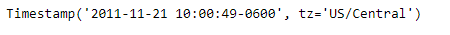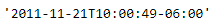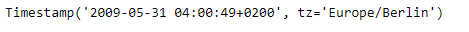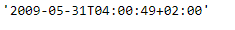# Python | Pandas Timestamp.isoformat

Python is a great language for doing data analysis, primarily because of the fantastic ecosystem of data-centric python packages. Pandas is one of those packages and makes importing and analyzing data much easier.

Pandas` Timestamp.isoformat()` function is used to convert the given Timestamp object into the ISO format.

Syntax :Timestamp.isoformat()

Parameters : None

Return : date time as a string

Example #1: Use `Timestamp.isoformat()` function to convert the date in the given Timestamp object to ISO format.

 `# importing pandas as pd ` `import` `pandas as pd ` ` `  `# Create the Timestamp object ` `ts ``=` `pd.Timestamp(year ``=` `2011``,  month ``=` `11``, day ``=` `21``, ` `                  ``hour ``=` `10``, second ``=` `49``, tz ``=` `'US/Central'``) ` ` `  `# Print the Timestamp object ` `print``(ts) `

Output :Now we will use the `Timestamp.isoformat()` function to convert the date in the given Timestamp object to ISO format.

 `# convert to ISO format ` `ts.isoformat() `

Output :As we can see in the output, the `Timestamp.isoformat()` function has returned the date in the ISO format.

Example #2: Use `Timestamp.isoformat()` function to convert the date in the given Timestamp object to ISO format.

 `# importing pandas as pd ` `import` `pandas as pd ` ` `  `# Create the Timestamp object ` `ts ``=` `pd.Timestamp(year ``=` `2009``, month ``=` `5``, day ``=` `31``, ` `                  ``hour ``=` `4``, second ``=` `49``, tz ``=` `'Europe/Berlin'``) ` ` `  `# Print the Timestamp object ` `print``(ts) `

Output :Now we will use the `Timestamp.isoformat()` function to convert the date in the given Timestamp object to ISO format.

 `# convert to ISO format ` `ts.isoformat() `

Output :As we can see in the output, the `Timestamp.isoformat()` function has returned the date in the ISO format.

My Personal Notes arrow_drop_upCheck out this Author's contributed articles.

If you like GeeksforGeeks and would like to contribute, you can also write an article using contribute.geeksforgeeks.org or mail your article to contribute@geeksforgeeks.org. See your article appearing on the GeeksforGeeks main page and help other Geeks.

Please Improve this article if you find anything incorrect by clicking on the "Improve Article" button below.

Article Tags :

Be the First to upvote.

Please write to us at contribute@geeksforgeeks.org to report any issue with the above content.